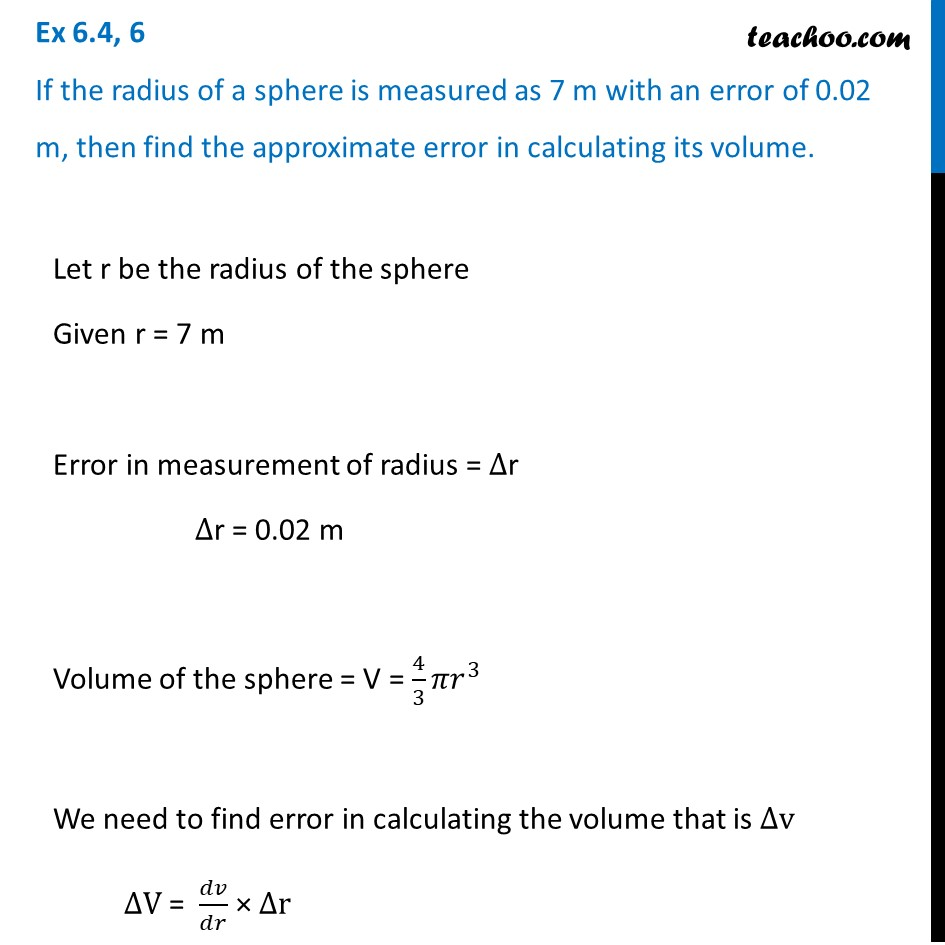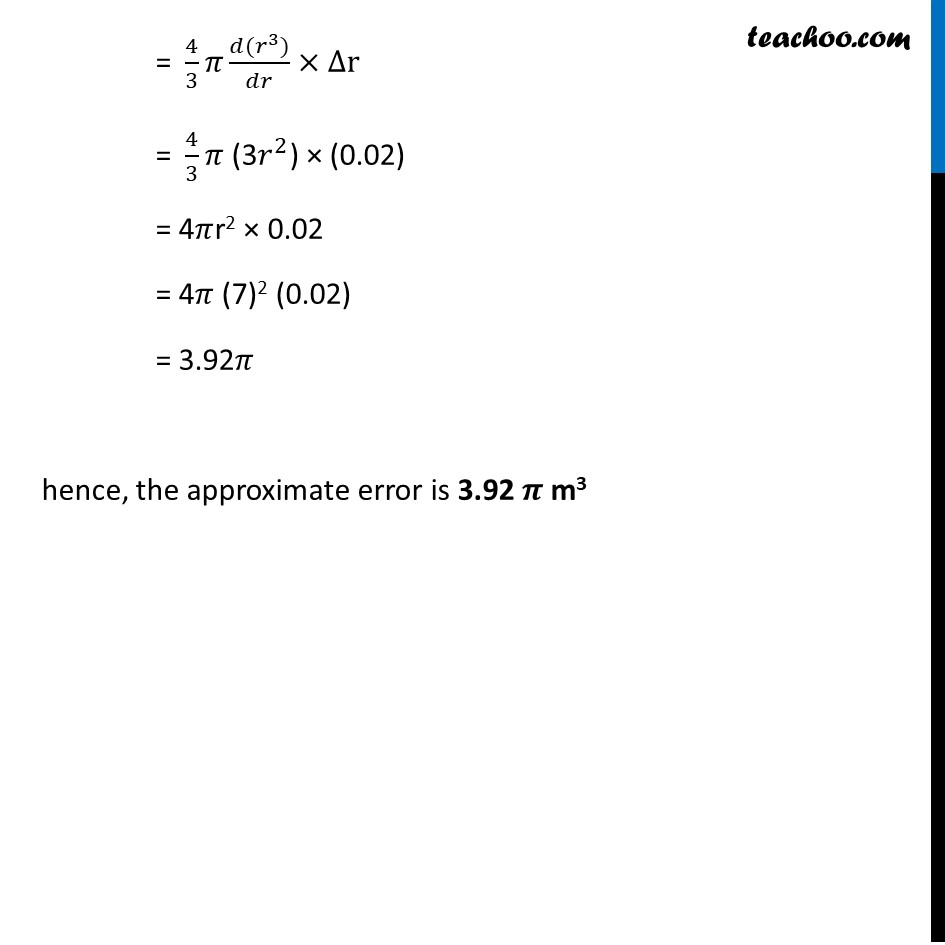Approximations (using Differentiation)

Chapter 6 Class 12 Application of Derivatives
Serial order wiseLearn in your speed, with individual attention - Teachoo Maths 1-on-1 Class

### Transcript

Question 6 If the radius of a sphere is measured as 7 m with an error of 0.02 m, then find the approximate error in calculating its volume.Let r be the radius of the sphere Given r = 7 m Error in measurement of radius = ∆r ∆r = 0.02 m Volume of the sphere = V = 4/3 𝜋𝑟^3 We need to find error in calculating the volume that is ∆v ∆V = 𝑑𝑣/𝑑𝑟 × ∆r = 𝑑(4/3 𝜋𝑟^3 )/𝑑𝑟 × ∆r = 4/3 𝜋 (𝑑(𝑟^3))/𝑑𝑟×∆r = 4/3 𝜋 (3𝑟^2) × (0.02) = 4𝜋r2 × 0.02 = 4𝜋 (7)2 (0.02) = 3.92𝜋 hence, the approximate error is 3.92 𝝅 m3